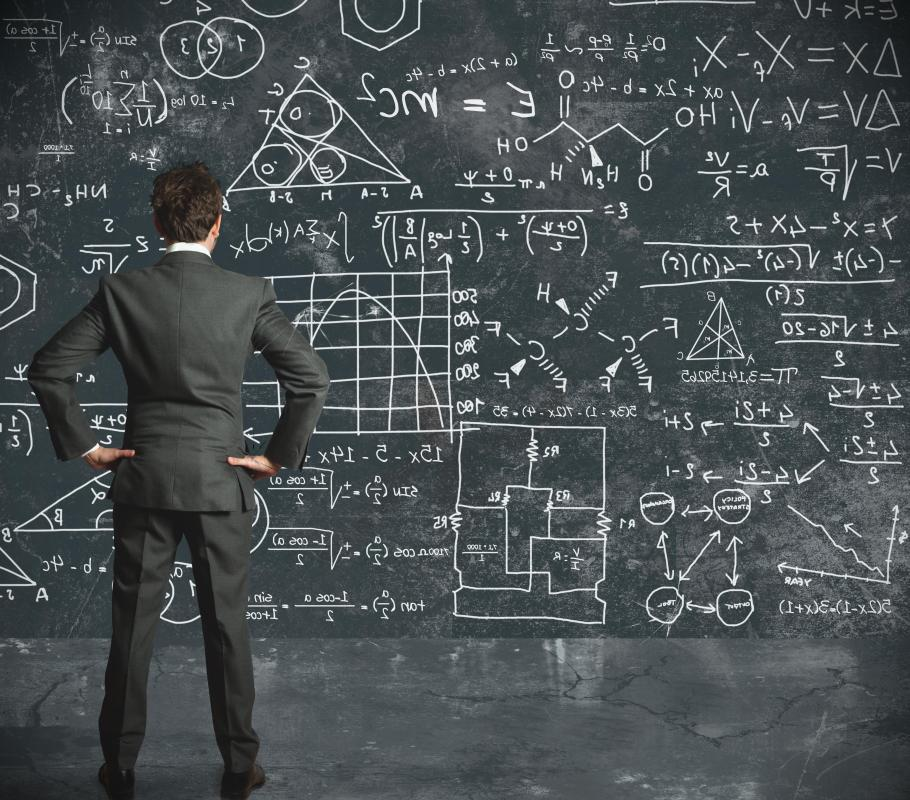# What Is the Magnetic Quantum Number? (with picture)

Helen Akers
Helen Akers

The magnetic quantum number is represented by the letter m or ml. This number is used to explain how an atom's electron is moving within one of its sub-particles. In magnetic quantum number physics, the number usually falls between -1 and 1.In order to identify the different orbits in an atom's sub-shell, the magnetic quantum number is used.

Each atom contains several electrons that are sometimes called sub-shells or particles. All of these particles move around each other. Each sub-particle moves in a fashion similar to how the solar system's planets orbit around the sun. It is a predefined pattern of movement that is controlled by chemical processes.

In order to identify the different orbits in an atom's sub-shell, the magnetic quantum number is used. Different forces, such as magnetic fields and gravity determine how atom particles move in relation to each other. The direction may be equated with a negative, neutral or positive number. For example, one atom may have three electrons, with one moving in a direction of -1, the other at 0, and yet another at 1.

These numbers are not always whole numbers. An atom may consist of a particle with a direction of 0.5. Both positive and negative charges are common within one atom. These opposite charges are what cause particles to bind to each other. The magnetic quantum number simply identifies which particles are moving in positive and negative directions.

There are three other quantum numbers involved in determining negative and positive energy of an atom besides the magnetic number. Together these numbers determine the energy state of the atom. A distinctive quantum condition exists inside each atom.

A spin magnetic quantum number determines the spin of an electron. While the magnetic number determines how an atom may shift its movement when under the influence of an external energy source, the spin magnetic quantum number reflects the strength of the atom's energy. This number also reflects where the atom falls on the negative and positive scale. In other words, it shows how far it is from either extreme or pole.

Momentum is reflected in quantum physics. In calculations it is expressed as L. The quantum number or m can be used to predict potential changes in the atom's movement along the axis of available negative and positive charges.

Essentially, the quantum number determines how much energy exits in atom. The calculations are used to determine how that energy might respond to outside forces. It can also predict movement and orientation changes within a specified range.

## You might also Like